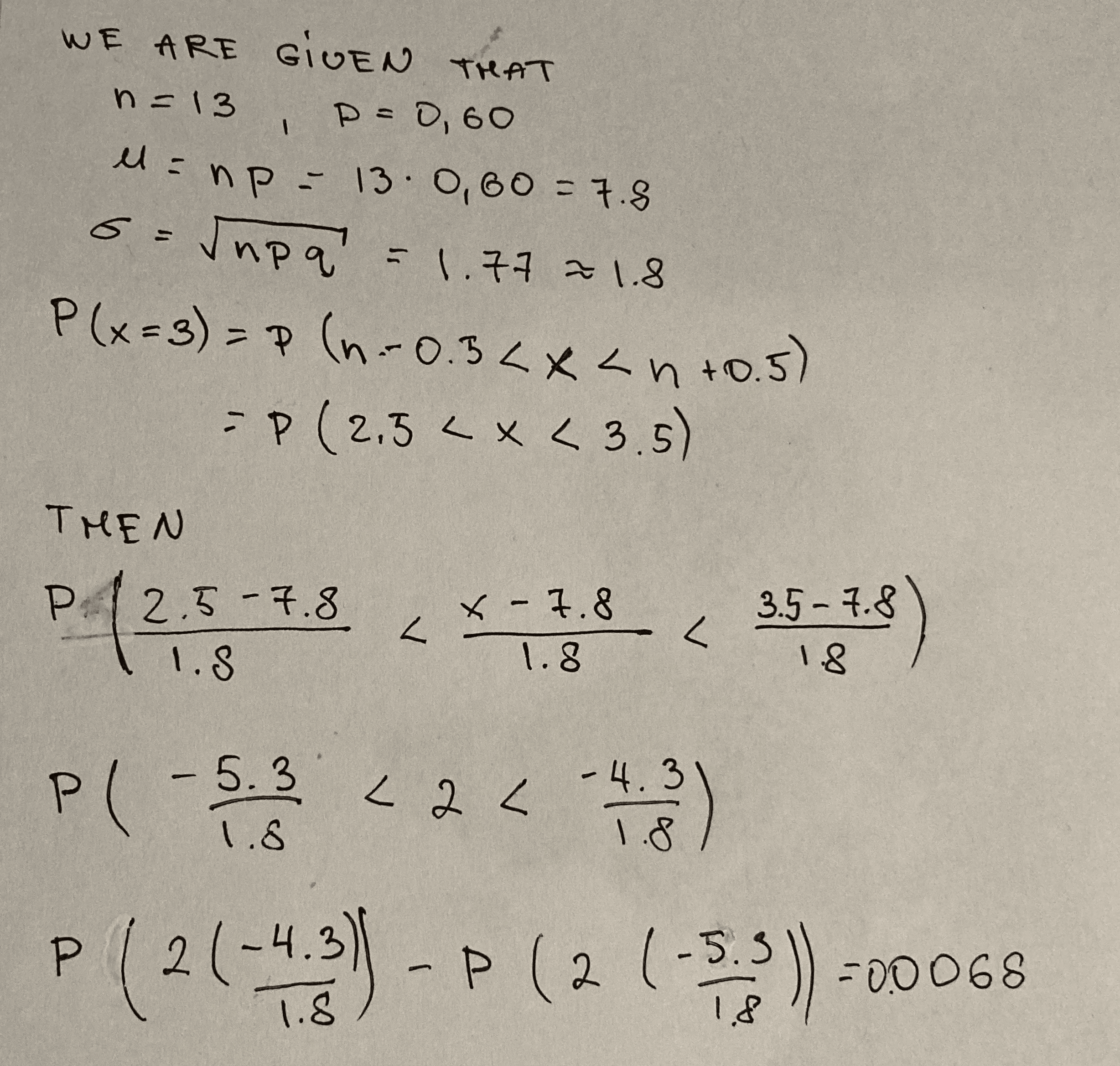Question# Find the normal approximation for the following binomial probability of P(x = 3) where n = 13 and p = 0.60

Binomial probability
ANSWEREDFind the normal approximation for the following binomial probability of $$P(x = 3)$$ where $$n = 13$$ and $$p = 0.60$$